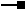# IGenerateStatistics Interface

## Description

IGenerateStatistics is used after cocreating a BaseStatistics object directly rather than getting one from IDataStatistics::Statistics. You populate the object with data using the AddValue method before returning statistics. Always set the SimpleStats property to True, unless you want frequency statistics (see IFrequencyStatistics).

## Members

Name DescriptionAddValue Adds a data value to the collection of values used to derive the statistics.FinalCompute May be called after all values have been added to establish frequeny table (the function is not required any more).Reset Clears out the currently gathered statistics.Sample Indicates if the statistics represent a sample of the data (used for calculating standard deviation).SimpleStats Indicates if simple statistics are sufficient. These are Count, Minimum, Maximum, Sum, Mean, Standard Deviation.

Adds a data value to the collection of values used to derive the statistics.

``````Public Sub AddValue ( _
ByVal Value As Double _
)
``````
``````public void AddValue (
double Value
);
``````

#### Description

The AddValue method adds the specified data value to the BaseStatistics. After adding all of the data values you can then generate statistics.

### IGenerateStatistics.FinalCompute Method

May be called after all values have been added to establish frequeny table (the function is not required any more).

``````Public Sub FinalCompute ( _
)
``````
``````public void FinalCompute (
);
``````

#### Description

The FinalCompute method is no longer required when generating statistics.

### IGenerateStatistics.Reset Method

Clears out the currently gathered statistics.

``````Public Sub Reset ( _
ByVal SimpleStats As Boolean _
)
``````
``````public void Reset (
bool SimpleStats
);
``````

#### Description

Removes all of the data values from the BaseStatistics.

### IGenerateStatistics.Sample Property

Indicates if the statistics represent a sample of the data (used for calculating standard deviation).

``````Public WriteOnly Property Sample
``````
``````public void Sample {set;}
``````

#### Description

Set Sample to True before calling IStatisticsResults::StandardDeviation if the BaseStatistics data values are a sample of the real data. Set Sample to False to retrieve the Population Standard Deviation with IStatisticsResults::StandardDeviation.

#### Remarks

By default IStatisticResults::StandardDeviation returns the Sample Standard Deviation, so there is no need to set the Sample property to True.

### IGenerateStatistics.SimpleStats Property

Indicates if simple statistics are sufficient. These are Count, Minimum, Maximum, Sum, Mean, Standard Deviation.

``````Public WriteOnly Property SimpleStats
``````
``````public void SimpleStats {set;}
``````

#### Description

Set SimpleStats to True to obtain statistics using the IStatisticsResults interface, and set SimpleStats to False to obtain statistics using the IFrequencyStatistics interface.

## Classes that implement IGenerateStatistics

Classes Description
BaseStatistics Base statistics class for generating and reporting statistics.